# ML | Classifying Data using an Auto-encoder

Prerequisites: Building an Auto-encoder

This article will demonstrate how to use an Auto-encoder to classify data. The data used below is the Credit Card transactions data to predict whether a given transaction is fraudulent or not. The data can be downloaded from here.

 `import` `pandas as pd  ` `import` `numpy as np ` `from` `sklearn.model_selection ``import` `train_test_split  ` `from` `sklearn.linear_model ``import` `LogisticRegression ` `from` `sklearn.svm ``import` `SVC ` `from` `sklearn.metrics ``import` `accuracy_score ` `from` `sklearn.preprocessing ``import` `MinMaxScaler  ` `from` `sklearn.manifold ``import` `TSNE ` `import` `matplotlib.pyplot as plt ` `import` `seaborn as sns ` `from` `keras.layers ``import` `Input``, Dense ` `from` `keras.models ``import` `Model, Sequential ` `from` `keras ``import` `regularizers `

 `# Changing the working location to the location of the data ` `cd C:\Users\Dev\Desktop\Kaggle\Credit Card Fraud ` ` `  `# Loading the dataset ` `df ``=` `pd.read_csv(``'creditcard.csv'``) ` ` `  `# Making the Time values appropriate for future work ` `df[``'Time'``] ``=` `df[``'Time'``].``apply``(``lambda` `x : (x ``/` `3600``) ``%` `24``) ` ` `  `# Separating the normal and fraudulent transactions ` `fraud ``=` `df[df[``'Class'``]``=``=` `1``] ` `normal ``=` `df[df[``'Class'``]``=``=` `0``].sample(``2500``) ` ` `  `# Reducing the dataset because of machinery constraints ` `df ``=` `normal.append(fraud).reset_index(drop ``=` `True``) ` ` `  `# Separating the dependent and independent variables ` `y ``=` `df[``'Class'``] ` `X ``=` `df.drop(``'Class'``, axis ``=` `1``) `

Step 3: Exploring the data

a)

 `df.head() `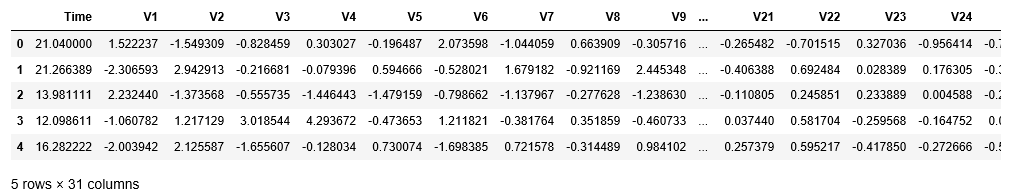b)

 `df.info() `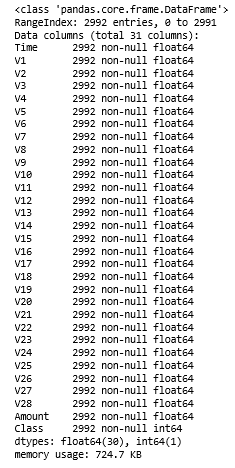c)

 `df.describe() `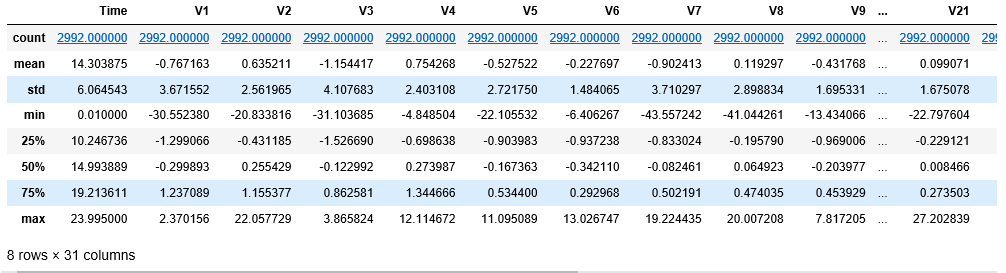Step 4: Defining a utility function to plot the data

 `def` `tsne_plot(x, y): ` `     `  `    ``# Setting the plotting background ` `    ``sns.``set``(style ``=``"whitegrid"``) ` `     `  `    ``tsne ``=` `TSNE(n_components ``=` `2``, random_state ``=` `0``) ` `     `  `    ``# Reducing the dimensionality of the data ` `    ``X_transformed ``=` `tsne.fit_transform(x) ` ` `  `    ``plt.figure(figsize ``=``(``12``, ``8``)) ` `     `  `    ``# Building the scatter plot ` `    ``plt.scatter(X_transformed[np.where(y ``=``=` `0``), ``0``],  ` `                ``X_transformed[np.where(y ``=``=` `0``), ``1``], ` `                ``marker ``=``'o'``, color ``=``'y'``, linewidth ``=``'1'``, ` `                ``alpha ``=` `0.8``, label ``=``'Normal'``) ` `    ``plt.scatter(X_transformed[np.where(y ``=``=` `1``), ``0``], ` `                ``X_transformed[np.where(y ``=``=` `1``), ``1``], ` `                ``marker ``=``'o'``, color ``=``'k'``, linewidth ``=``'1'``, ` `                ``alpha ``=` `0.8``, label ``=``'Fraud'``) ` ` `  `    ``# Specifying the location of the legend ` `    ``plt.legend(loc ``=``'best'``) ` `     `  `    ``# Plotting the reduced data ` `    ``plt.show() `

Step 5: Visualizing the original data

 `tsne_plot(X, y) `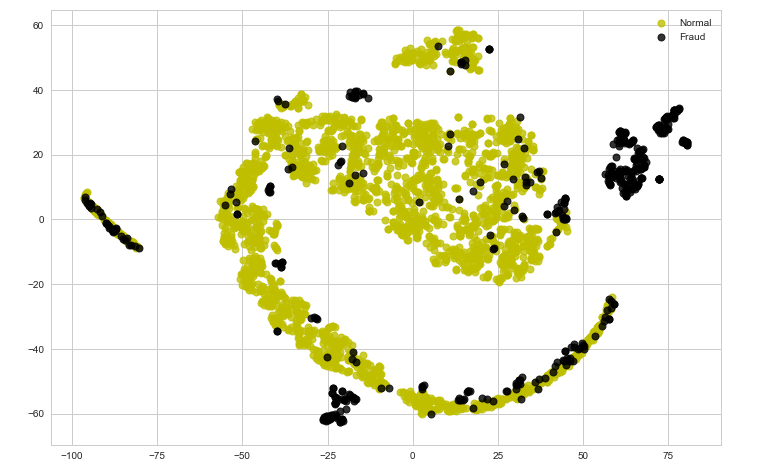Note that the data currently is not easily separable. In the following steps, we will try to encode the data using an Auto-encoder and analyze the results.

Step 6: Cleaning the data to make it suitable for the Auto-encoder

 `# Scaling the data to make it suitable for the auto-encoder ` `X_scaled ``=` `MinMaxScaler().fit_transform(X) ` `X_normal_scaled ``=` `X_scaled[y ``=``=` `0``] ` `X_fraud_scaled ``=` `X_scaled[y ``=``=` `1``] `

Step 7: Building the Auto-encoder neural network

 `# Building the Input Layer ` `input_layer ``=` `Input``(shape ``=``(X.shape[``1``], )) ` ` `  `# Building the Encoder network ` `encoded ``=` `Dense(``100``, activation ``=``'tanh'``, ` `                ``activity_regularizer ``=` `regularizers.l1(``10e``-``5``))(input_layer) ` `encoded ``=` `Dense(``50``, activation ``=``'tanh'``, ` `                ``activity_regularizer ``=` `regularizers.l1(``10e``-``5``))(encoded) ` `encoded ``=` `Dense(``25``, activation ``=``'tanh'``, ` `                ``activity_regularizer ``=` `regularizers.l1(``10e``-``5``))(encoded) ` `encoded ``=` `Dense(``12``, activation ``=``'tanh'``, ` `                ``activity_regularizer ``=` `regularizers.l1(``10e``-``5``))(encoded) ` `encoded ``=` `Dense(``6``, activation ``=``'relu'``)(encoded) ` ` `  `# Building the Decoder network ` `decoded ``=` `Dense(``12``, activation ``=``'tanh'``)(encoded) ` `decoded ``=` `Dense(``25``, activation ``=``'tanh'``)(decoded) ` `decoded ``=` `Dense(``50``, activation ``=``'tanh'``)(decoded) ` `decoded ``=` `Dense(``100``, activation ``=``'tanh'``)(decoded) ` ` `  `# Building the Output Layer ` `output_layer ``=` `Dense(X.shape[``1``], activation ``=``'relu'``)(decoded) `

Step 8: Defining and Training the Auto-encoder

 `# Defining the parameters of the Auto-encoder network ` `autoencoder ``=` `Model(input_layer, output_layer) ` `autoencoder.``compile``(optimizer ``=``"adadelta"``, loss ``=``"mse"``) ` ` `  `# Training the Auto-encoder network ` `autoencoder.fit(X_normal_scaled, X_normal_scaled,  ` `                ``batch_size ``=` `16``, epochs ``=` `10``,  ` `                ``shuffle ``=` `True``, validation_split ``=` `0.20``) `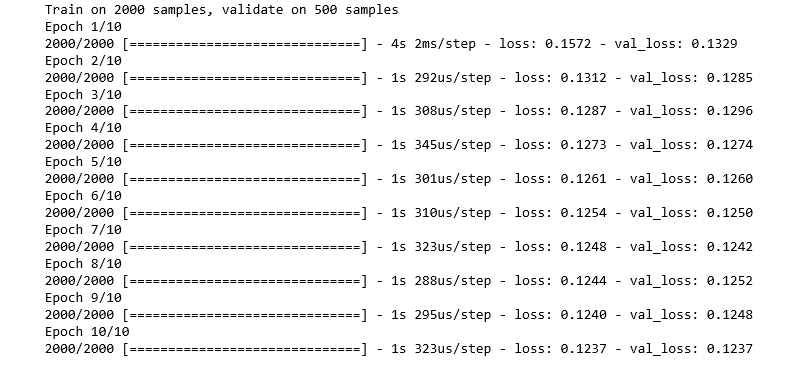Step 9: Retaining the encoder part of the Auto-encoder to encode data

 `hidden_representation ``=` `Sequential() ` `hidden_representation.add(autoencoder.layers[``0``]) ` `hidden_representation.add(autoencoder.layers[``1``]) ` `hidden_representation.add(autoencoder.layers[``2``]) ` `hidden_representation.add(autoencoder.layers[``3``]) ` `hidden_representation.add(autoencoder.layers[``4``]) `

Step 10: Encoding the data and visualizing the encoded data

 `# Separating the points encoded by the Auto-encoder as normal and fraud ` `normal_hidden_rep ``=` `hidden_representation.predict(X_normal_scaled) ` `fraud_hidden_rep ``=` `hidden_representation.predict(X_fraud_scaled) ` ` `  `# Combining the encoded points into a single table  ` `encoded_X ``=` `np.append(normal_hidden_rep, fraud_hidden_rep, axis ``=` `0``) ` `y_normal ``=` `np.zeros(normal_hidden_rep.shape[``0``]) ` `y_fraud ``=` `np.ones(fraud_hidden_rep.shape[``0``]) ` `encoded_y ``=` `np.append(y_normal, y_fraud) ` ` `  `# Plotting the encoded points ` `tsne_plot(encoded_X, encoded_y) `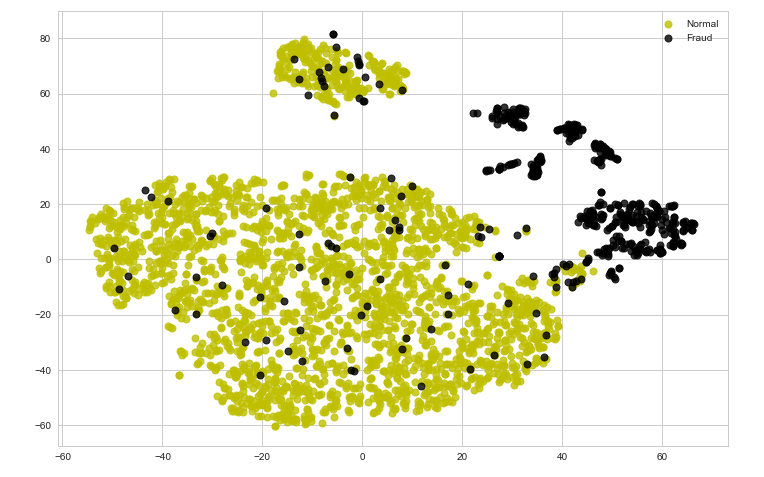Observe that after encoding the data, the data has come closer to being linearly separable. Thus in some cases, encoding of data can help in making the classification boundary for the data as linear. To analyze this point numerically, we will fit the Linear Logistic Regression model on the encoded data and the Support Vector Classifier on the original data.

Step 11: Splitting the original and encoded data into training and testing data

 `# Splitting the encoded data for linear classification ` `X_train_encoded, X_test_encoded, y_train_encoded, y_test_encoded ``=` `train_test_split(encoded_X, encoded_y, test_size ``=` `0.2``) ` ` `  `# Splitting the original data for non-linear classification ` `X_train, X_test, y_train, y_test ``=` `train_test_split(X, y, test_size ``=` `0.2``) `

Step 12: Building the Logistic Regression model and evaluating it’s performance

 `# Building the logistic regression model ` `lrclf ``=` `LogisticRegression() ` `lrclf.fit(X_train_encoded, y_train_encoded) ` ` `  `# Storing the predictions of the linear model ` `y_pred_lrclf ``=` `lrclf.predict(X_test_encoded) ` ` `  `# Evaluating the performance of the linear model ` `print``(``'Accuracy : '``+``str``(accuracy_score(y_test_encoded, y_pred_lrclf))) `Step 13: Building the Support Vector Classifier model and evaluating it’s performance

 `# Building the SVM model ` `svmclf ``=` `SVC() ` `svmclf.fit(X_train, y_train) ` ` `  `# Storing the predictions of the non-linear model ` `y_pred_svmclf ``=` `svmclf.predict(X_test) ` ` `  `# Evaluating the performance of the non-linear model ` `print``(``'Accuracy : '``+``str``(accuracy_score(y_test, y_pred_svmclf))) `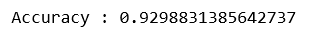Thus the performance metrics support the point stated above that encoding the data can sometimes be useful for making a data linearly separable as the performance of the Linear Logistic Regression model is very close to the performance of the Non-Linear Support Vector Classifier model.

My Personal Notes arrow_drop_upCheck out this Author's contributed articles.

If you like GeeksforGeeks and would like to contribute, you can also write an article using contribute.geeksforgeeks.org or mail your article to contribute@geeksforgeeks.org. See your article appearing on the GeeksforGeeks main page and help other Geeks.

Please Improve this article if you find anything incorrect by clicking on the "Improve Article" button below.

Improved By : shubham_singh

Article Tags :
Practice Tags :

Be the First to upvote.

Please write to us at contribute@geeksforgeeks.org to report any issue with the above content.